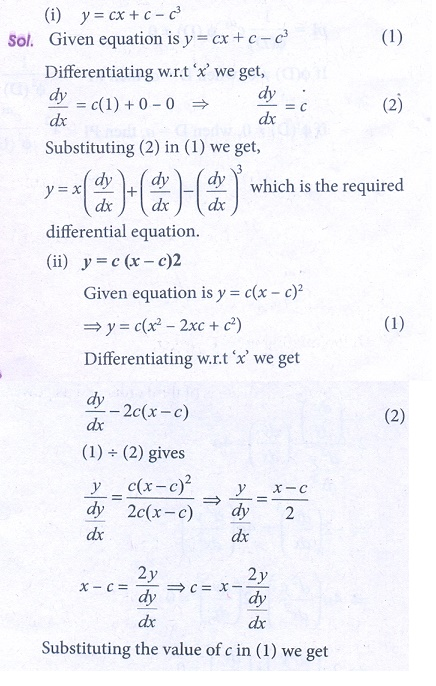Home | | Business Maths 12th Std | Exercise 4.1: Formation of ordinary differential equations

# Exercise 4.1: Formation of ordinary differential equations

Book back answers and solution for Exercise questions - Maths: Differential Equations : Formation of ordinary differential equations: Solved Example Problems with Answers, Solution

Exercise 4.1

1. Find the order and degree of the following differential equations.2.   Find the differential equation of the following

(i) y = cx + c  c3   (ii) y = c ( x  c)2   (iii) xy = c2   (iv) x 2 + y2 = a23.  Form the differential equation by eliminating α and β (x-α)2+(y-β)2 = r24. Find the differential equation of the family of all straight lines passing through the origin.5. Form the differential equation that represents all parabolas each of which has a latus rectum 4a and whose axes are parallel to the x axis.6. Find the differential equation of all circles passing through the origin and having their centers on the y axis.7. Find the differential equation of the family of parabola with foci at the origin and axis along the x-axis.Tags : Problem Questions with Answer, Solution , 12th Business Maths and Statistics : Chapter 4 : Differential Equations
Study Material, Lecturing Notes, Assignment, Reference, Wiki description explanation, brief detail
12th Business Maths and Statistics : Chapter 4 : Differential Equations : Exercise 4.1: Formation of ordinary differential equations | Problem Questions with Answer, Solution